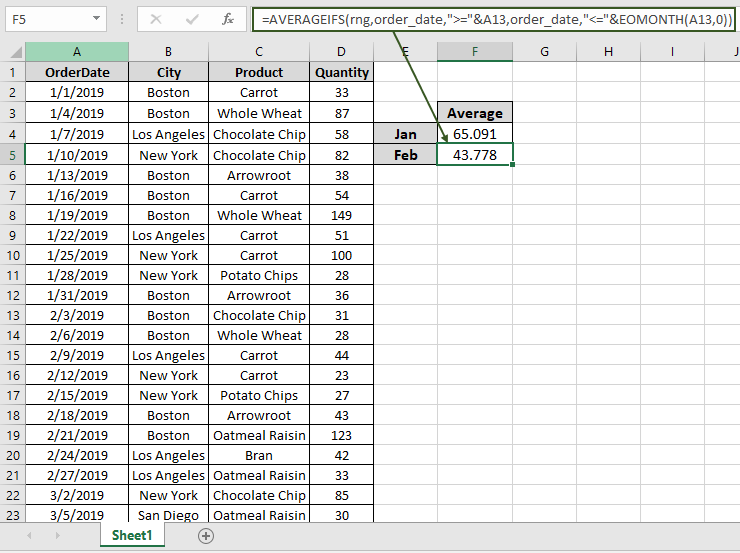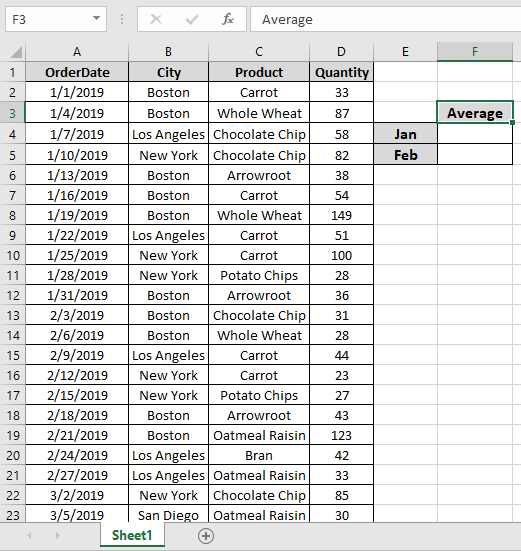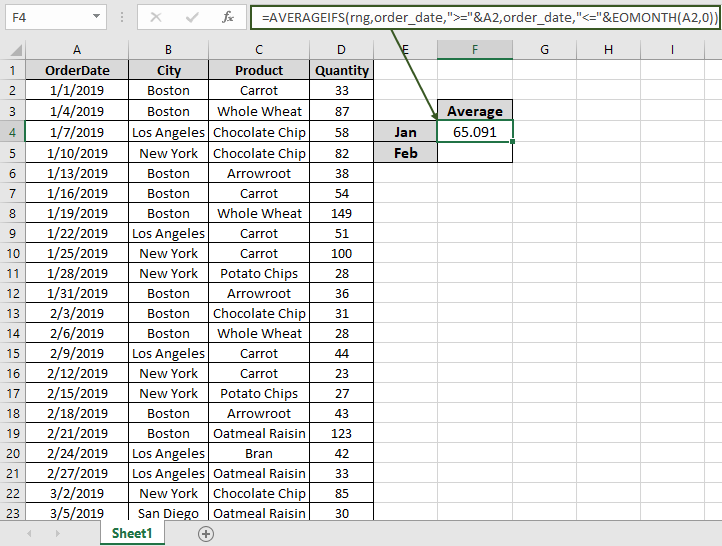# How to Get Average if Matches MonthIn this article, we will learn how to get the average of matched months only in Excel.

For instance, you have a large list of data and you need to find the Average of the numbers in a particular month.

For this article we will be needing the use of the following functions:

1. AVERAGEIFS Function
2. EOMONTH function

Excel AVERAGEIFS function is used to find the average of the array based on multiple criteria or conditions.

The EOMONTH function is used to find the last day of the given month.

Now we will make a formula out of these functions. Here we will be given the data and we needed to find the AVERAGE of the numbers in a particular month.

Now we will make a formula out of these functions. Here we will be given the data and we need to find the AVERAGE of the numbers in a particular month.
Use the formula:

= AVERAGEIFS ( rng , order_date , ">=" & date , order_date , "<=" & EOMONTH( date , 0 ) )
• EOMONTH function returns at the end of the month date of the date given as an argument.
• AVERAGEIFS  Funciton takes the average of the numbers between having a date greater than the given date and less than the end of the month date.

Note: Do not provide data directly to the function. Use DATE function or use cell reference for date argument in excel as Excel reads date only with the correct order.

Let's test this formula via running it on an example

Here we have data in range A1:D51. we need to find the average of quantity of the month of January.First we will find a date which has the first date of the month which is the A2 cell. Named ranges are rng ( D2 : D51 ) and order_date ( A2 : A51).

Use the Formula:

= AVERAGEIFS ( rng , order_date , ">=" & date , order_date , "<=" & EOMONTH( date , 0 ) )

Explanation:

• EOMONTH (A2, 0) returns the 31st January as the last date.
• ">=" & A2 returns a criteria that the value greater than date in A2.
• "<=" & EOMONTH ( A2 , 0 ) ) returns a criteria that the value less than the end of the month date.
• AVERAGEIFS takes all the above arguments and returns the average of the number given below.

{ 33 ; 87 ; 58 ; 82 ; 38 ; 54 ; 149 ; 51 ; 100 ; 28 ; 36 }

Here the A2 is given as cell reference & Named ranges given as rng ( D2 : D51 ) and order_date ( A2 : A51).As you can see in the above snapshot the AVERAGE of the quantity in January comes out to be 65.091 .

Now we will get the AVERAGE of the quantity in February by changing the First date argument of the function

Use the Formula:

= AVERAGEIFS ( rng , order_date , ">=" & A13 , order_date , "<=" & EOMONTH ( A13 , 0 ) )As you can see in the above snapshot the AVERAGE of the quantity in January comes out to be 43.778 .

Notes:

1.  The function returns #VALUE! error if the argument to the function is non-numeric.
2. Do not provide date directly to the function. Use DATE function or use cell reference for date argument in excel as Excel reads date only with the correct order.

Hope this article about how to AVERAGE IF with months in Excel is explanatory. Find more articles on SUMPRODUCT functions here. Please share your query below in the comment box. We will assist you.

Related Articles

How to use the AVERAGEIFS function in excel

How to use the SUM function in excel

How to use the ISFORMULA function in Excel

How to use the ROWS function in Excel

Validation of text entries

Create drop down list in excel with colour

Popular Articles

Edit a dropdown list

Absolute reference in Excel

If with conditional formatting

If with wildcards

Vlookup by date

Join first and last name in excel

Count cells which match either A or B

Convert Inches To Feet and Inches in Excel 2016

50 Excel Shortcuts to Increase Your Productivity

Terms and Conditions of use

The applications/code on this site are distributed as is and without warranties or liability. In no event shall the owner of the copyrights, or the authors of the applications/code be liable for any loss of profit, any problems or any damage resulting from the use or evaluation of the applications/code.